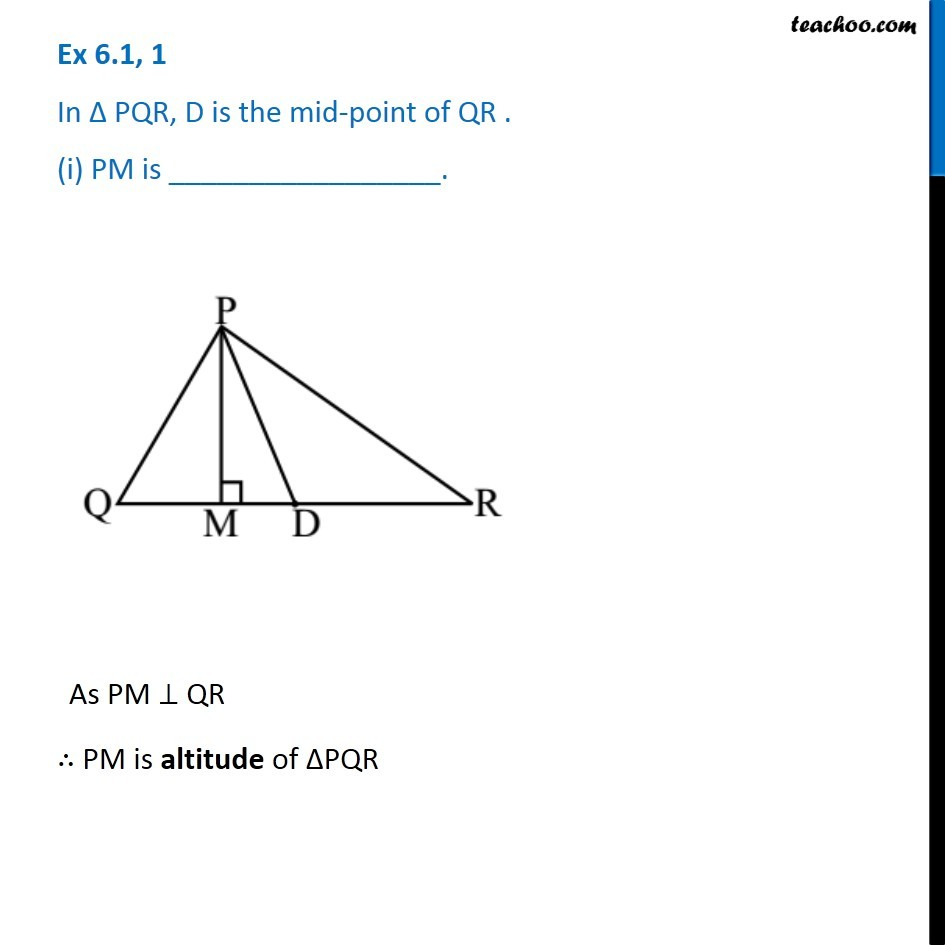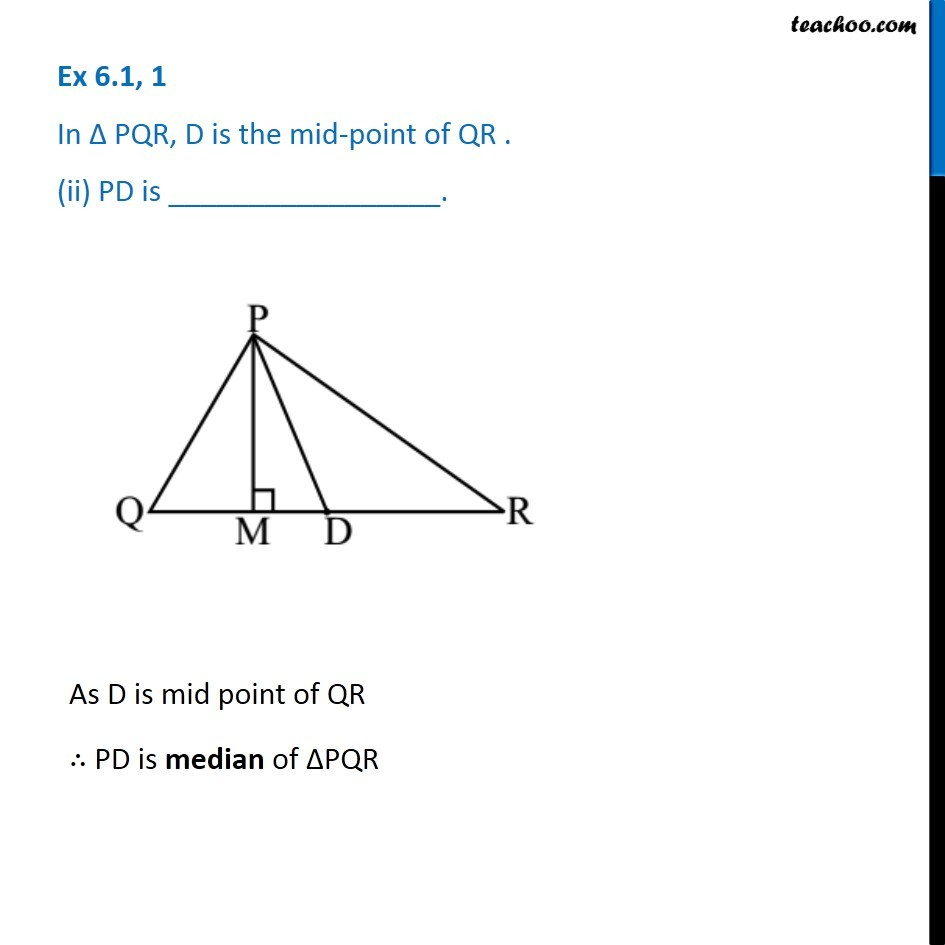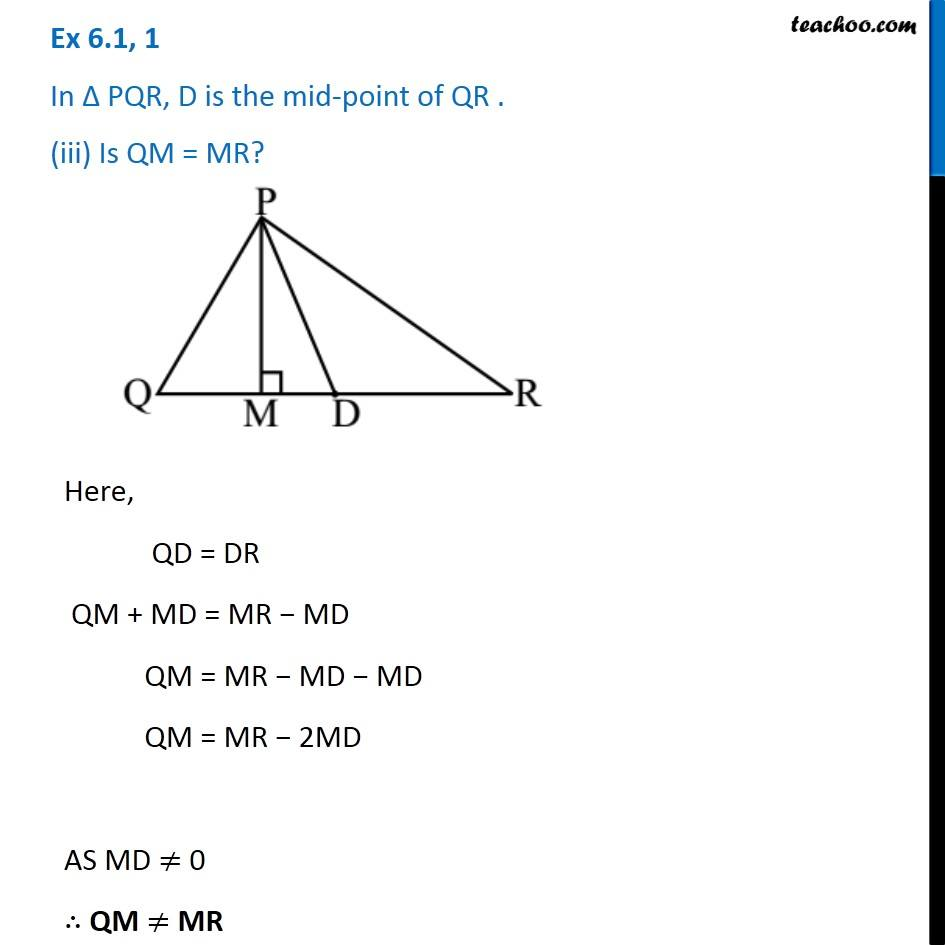1. Chapter 6 Class 7 Triangle and its Properties
2. Serial order wise
3. Ex 6.1

Transcript

Ex 6.1, 1 In ∆ PQR, D is the mid-point of QR . (i) PM is _________________. As PM ⊥ QR ∴ PM is altitude of ∆PQR Ex 6.1, 1 In ∆ PQR, D is the mid-point of QR . (ii) PD is _________________. As D is mid point of QR ∴ PD is median of ∆PQR Ex 6.1, 1 In ∆ PQR, D is the mid-point of QR . (iii) Is QM = MR? Here, QD = DR QM + MD = MR − MD QM = MR − MD − MD QM = MR − 2MD AS MD ≠ 0 ∴ QM ≠ MR

Ex 6.1